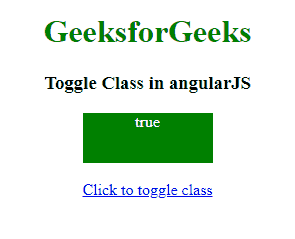# How to toggle class using AngularJS ?

• Last Updated : 04 Aug, 2022

In this article, we will toggle the class of an element with the help of AngularJS. The toggling of the class can be done in 2 ways, i.e., either specifying the value to 0 or 1, depending upon the condition satisfied, by initially defining the value as 0, or depending on the boolean value, i.e., true or false, with satisfying the required condition, by initially setting the value as true.

Approach 1:

• In this example, when a button is clicked then the class of an element is changed.
• So, a function is called when the button is clicked.
• That function toggles the class from val to !val(means 0 to 1 and vice-versa).
• In the function called, we simply check if it is class1 then change it to class2 else do the opposite.

Example: This example describes the toggling of the class in AngularJS by specifying the value in the terms of 0 & 1.

## HTML

 ```<``html``>` `<``head``>``    ``<``script` `src``=``"https://ajax.googleapis.com/ajax/libs/angularjs/1.2.13/angular.min.js"``>``    ````    ``<``script``>``        ``var myApp = angular.module("app", []);``        ``myApp.controller("controller", function(\$scope) {``            ``\$scope.val = 0;``            ``\$scope.toggleClass = function(sel) {``                ``if(\$scope.val == 0) {``                    ``\$scope.val = 1;``                ``} else {``                    ``\$scope.val = 0;``                ``}``            ``};``        ``});``    ````    ``<``style``>``        ``.class1 {``            ``color: white;``            ``background: blue;``        ``}``        ` `        ``.class2 {``            ``color: white;``            ``background: green;``        ``}``    ````` `<``body` `style``=``"text-align:center;"``>``    ``<``h1` `style``=``"color:green;"``>``        ``GeeksforGeeks``    ````    ``<``h3``>``        ``Toggle Class in angularJS``    ````    ``<``div` `ng-app``=``"app"``>``        ``<``div` `ng-controller``=``"controller"``>``            ``<``div` `ng-class="{'class1':!val,``                ``'class2': val}">``                ``{{ val }}``            ````            ``<``br``>``            ``<``a` `href``=``"javascript:void(0);"``               ``ng-click``=``'toggleClass();'``>``                ``Click to toggle class``            ````        ````    ```````

Output:Approach 2:

• This example is somewhat similar to the previous one but used boolean values in place of 0 and 1.
• So, a function is called when the button is clicked.
• That function toggles the class from val to !val(means true to false and vice-versa).
• In the function called, we simply check if it is class1 then change it to class2 else do the opposite.

Example: This example describes toggling the class in AngularJS by specifying the boolean value.

## HTML

 ```<``html``>` `<``head``>``    ``<``script` `src``=``"https://ajax.googleapis.com/ajax/libs/angularjs/1.2.13/angular.min.js"``>``    ````    ``<``script``>``        ``var myApp = angular.module("app", []);``        ``myApp.controller("controller", function(\$scope) {``            ``\$scope.val = true;``            ``\$scope.toggleClass = function(sel) {``                ``if(\$scope.val == true) {``                    ``\$scope.val = false;``                ``} else {``                    ``\$scope.val = true;``                ``}``            ``};``        ``});``    ````    ``<``style``>``        ``.class1 {``            ``color: white;``            ``background: blue;``        ``}``        ` `        ``.class2 {``            ``color: white;``            ``background: green;``        ``}``        ` `        ``#div {``            ``height: 50px;``            ``width: 130px;``            ``color: white;``            ``margin: 0 auto;``        ``}``    ````` `<``body` `style``=``"text-align:center;"``>``    ``<``h1` `style``=``"color:green;"``>``        ``GeeksforGeeks``    ````    ``<``h3``>``        ``Toggle Class in angularJS``    ````    ``<``div` `ng-app``=``"app"``>``        ``<``div` `ng-controller``=``"controller"``>``            ``<``div` `id``=``'div'` `ng-class="{'class1':!val,``                ``'class2': val}">``                ``{{ val }}``             ````            ``<``br``>``            ``<``a` `href``=``"javascript:void(0);"``               ``ng-click``=``'toggleClass();'``>``                ``Click to toggle class``            ````        ````    ```````

Output:My Personal Notes arrow_drop_up